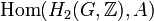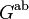# Formula for second cohomology group for trivial group action in terms of Schur multiplier and abelianization

Jump to: navigation, search

## Formula

Suppose$G$ is a group and$A$ is an abelian group. Then, the second cohomology group for trivial group action$H^2(G;A)$ occurs in the following natural short exact sequence (this sequence arises as the$n = 2$ case of the dual universal coefficient theorem for group cohomology):$0 \to \operatorname{Ext}^1_{\mathbb{Z}}(G^{\operatorname{ab}},A) \to H^2(G;A) \to \operatorname{Hom}(H_2(G;\mathbb{Z}),A) \to 0$

The sequence splits (though the splitting is not necessarily natural), and we get:$H^2(G;A) \cong \operatorname{Hom}(H_2(G,\mathbb{Z}),A) \oplus \operatorname{Ext}^1_{\mathbb{Z}}(G^{\operatorname{ab}},A)$

Here:

•$\operatorname{Hom}(H_2(G,\mathbb{Z}),A)$, also called the second cohomology group up to isoclinism, is the group (under pointwise addition) of group homomorphisms from$H_2(G;\mathbb{Z})$ to$A$.$H_2(G;\mathbb{Z})$ is the second homology group for trivial group action, and is also called the Schur multiplier of$G$. It is alternatively denoted as$M(G)$.
•$\operatorname{Ext}^1_{\mathbb{Z}}(G^{\operatorname{ab}},A)$ describes abelian group extensions with normal subgroup$A$ and quotient group$G^{\operatorname{ab}}$.

## Interpretation of left map of the sequence

The map:$\operatorname{Ext}^1_{\mathbb{Z}}(G^{\operatorname{ab}},A) \to H^2(G;A)$

takes an abelian group extension with normal subgroup$A$ and quotient group$G^{\operatorname{ab}}$, and gives an extension with$G$ on top of$A$ as follows: the restriction to the derived subgroup$[G,G]$ splits and the quotient acts as per the element of$\operatorname{Ext}^1_{\mathbb{Z}}(G^{\operatorname{ab}},A)$. Explicitly, it can be thought of as a composite of two maps:$\operatorname{Ext}^1_{\mathbb{Z}}(G^{\operatorname{ab}},A) \to H^2(G^{\operatorname{ab}};A) \to H^2(G;A)$

where the first map treats an abelian group extension simply as a group extension, and the second map uses the contravariance of$H^2$ in its first argument.

## Interpretation of right map of the sequence

The description can be understood better if we consider the following two short exact sequences. Because the sequences involve possibly non-abelian groups on the quotient side, we denote the trivial group at the right end of these short exact sequences as 1 rather than 0. We have:$0 \to M(G) \to G \wedge G \to [G,G] \to 1$

and consider the sequence corresponding to an extension group$E$ that in turn corresponds to an element of$H^2(G;A)$:$0 \to A \to E \to G \to 1$

Now, the commutator map in$E$ gives a map$G \wedge G \to [E,E]$ because$A$ is central (see commutator map is homomorphism from exterior square to derived subgroup). Composing with the inclusion of$[E,E]$ in$E$, we get a map$G \wedge G \to E$. We also have the usual inclusion map$[G,G] \to G$. The following diagram commutes:$\begin{array}{ccccccccc} 0 & \to & M(G) & \to & G \wedge G & \to & [G,G] & \to & 1\\ \downarrow && && \downarrow &&\downarrow&& \downarrow\\ 0 & \to & A & \to & E & \to & G & \to & 1\\ \end{array}$

We can construct a unique map$M(G) \to A$ such that the diagram continues to be commutative, and that is the map we seek.$\begin{array}{ccccccccc} 0 & \to & M(G) & \to & G \wedge G & \to & [G,G] & \to & 1\\ \downarrow && \downarrow && \downarrow && \downarrow&& \downarrow\\ 0 & \to & A &\to & E & \to & G & \to & 1\\ \end{array}$

## Facts used

1. Dual universal coefficient theorem for group cohomology
2. First homology group for trivial group action equals tensor product with abelianization: In particular,$H_1(G;\mathbb{Z})$ is just$G^{\operatorname{ab}}$.
3. Schur multiplier is kernel of commutator map homomorphism from exterior square to derived subgroup

## Proof

The proof for general$G$ follows by combining facts (1) and (2). The further simplification and interpretation follows by fact (3). Actually, there's more work that needs to be done than just quoting these facts, because we have to show that all the different descriptions give the same map.